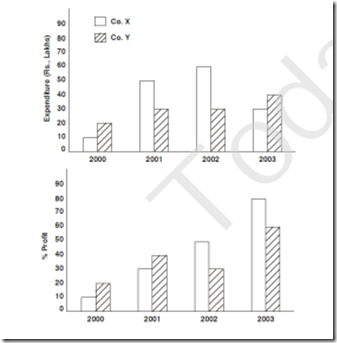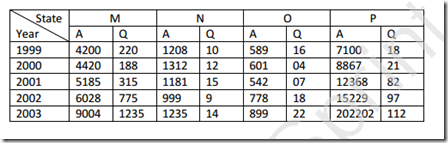Now you can Subscribe using RSS

# IBPS PO 2017 Quantitative aptitude Practice Quiz 4

IBPS EXAM Guru

1 .

Direction (Q. 1 to 5): Study the 2 graphs and answer the questions based on them:
Use formula:-
% Profit = $Income - Expenditure\over Expenditure$ x 100$Q.$ Approximately what % is the income of company X in 2001 less than that of company Y in 2003?

1.$100\over67$
2.$1\over64$
3.$64\over65$
4.None of the above

 A.   1 B.   2 C.   3 D.   4
2 . In which years is the income of company X more than that of company Y?

 A.   1 B.   2 C.   3 D.   4
3 . What is the average income of company X over the 4-year period? (Rs lakhs)

 A.   50 B.   54 C.   55 D.   61
4 . In the year in which company X had maximum income, what was the income of company Y. (Rs lakhs)

 A.   39 B.   40 C.   41 D.   42
5 . The ratio of income of company Y in 2000 to that of company X in 2003 is:

1.$1\over3$
2.$4\over7$
3.$3\over7$
4.$4\over9$

 A.   1 B.   2 C.   3 D.   4
6 .

Direction (Q. 6 to 10): In these questions, first study the information provided in the table and then answers the following questions.Table shows Bank P.O. selections from 4 States M, N, O, P in past 5 years, in written exams

$Q.$What is the approximate % of number of candidates who appeared from States N and O in 2002 to the candidates appeared from P in 1999?

 A.   24% B.   25% C.   26% D.   22%
7 . What % of candidates qualified the written exams in 2000?

 A.   1.5% B.   2% C.   1.8% D.   2.4%
8 . The number of candidates qualifying from State M bears what ratio to those qualifying from State N?

1.$250\over9$
2.$201\over17$
3.$120\over11$
4.$125\over3$

 A.   1 B.   2 C.   3 D.   4
9 . Which State had the best results in 2003?

 A.   O B.   P C.   M D.   N
10 . When was the result of State Q its best?

 A.   1999 B.   2000 C.   2001 D.   2002
1 .
 Answer : Option D Explanation : X’s income (2001) = $130\over 100$ x 50 = Rs 65 lakh and Y’s income (2003) = $160\over 100$ x 40 = Rs 64 lakh - x > y
2 .
 Answer : Option B Explanation : The income of A and B are as follows:2000: x = $110\over100$ x 10 = 11 lakhY = $120\over100$ x 20 = 24 lakh - x < y2001: x = $130 \times 50\over 100$ = 65 lakhY = $140\over100$x 30 = 42 lakh - x > y 2002: x =$150 \times 60\over 100$ = 90 lakhY = $130\over100$x 30 = 39 lakh - x > y 2003: x =$180 \times 30\over 100$ = 54 lakhY = $160\over100$x 40 = 64 lakh - x < y Thus, 2 years (2001, 2002).
3 .
 Answer : Option C Explanation : From the same table above, required average = $11+65+90+54\over4$ = $200\over4$ = Rs 55 lakh
4 .
 Answer : Option A Explanation : From the same table above, x has maximum income of Rs 90 lakh, in 2002.
5 .
 Answer : Option D Explanation : Required ratio = $24\over 54$ = $12\over 27$ = $4\over9$
6 .
 Answer : Option B Explanation : Required % = $999+778\over7100$ x 100 = $1\over4$ or 25%
7 .
 Answer : Option A Explanation : Required % = $188+12+4+21\over4420+1312+601+8867$ x 100= $225\over15,000$ x 100 = 1.5%
8 .
 Answer : Option D Explanation : Ratio = $220+188+315+775+1002\over10+12+15+9+14$= $2500\over60$ = $125\over3$
9 .
 Answer : Option C Explanation : Clearly, M has highest pass ratio = $1002\over9004$
10 .
 Answer : Option A Explanation : Highest pass ratio = 3%